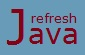# What is operator in Java

In computer programming languages, the definitions of operator and operand are almost the same as in mathematics. An operator is basically a special symbol which tells the computer to perform specific operation on one, two or three operands and then return a result.

### What is operand in Java ?

An operand is simply a value on which the operation is performed. This value can be in the form of constant, variable, expression or any method which returns a value. For example in expression `2+3, 2` and `3` are operands and `+` is an operator that performs addition operation. Similarly in expression `a+b`, `a` and `b` are operands and `+` is an operator.

### What is an expression in java

An expression is a construct made up of variables, operators and method invocations, these expressions are constructed as per the syntax of the programming language and evaluates to a single value. For example `a+b, a+b*c, a = 10, c = a + b, a > b, sqrt(4), (a+b)*(c-d)` etc. An expression may contain sub-expressions within it.

## Different types of operators in Java

There are following types of operators in java, you can refer the specific tutorial of these operator types to see the details like what all operators are there in that type, what they does and their example programs.

Operators can also be categorized by the number of operands they operate on. Most operator requires one or two operands except ternary operator which requires three operands. So we can categorize above operators in three types as well :

• Unary operator
• Binary operator
• Ternary operator

### What is unary operator in java ?

An operator which operates on one operand is known as unary operator. For example `+, -, ++` etc are unary operators, usage example `+2, -3, ++a` etc.

### What is binary operator in java ?

An operator which operates on two operands is known as binary operator. For example `+, -, *` etc are binary operators, usage example `2+3, 5-3, a*b` etc.

### What is ternary operator in java ?

An operator which operates on three operands is known as ternary operator. There is only one ternary operator in java which is `?:`, also known as `if then else` operator.

## Java operators list

The table below shows the list of all operators in java which you can use in your programs. For this table let's assume we have operands or variables `a, b` and `c`.

Operator Symbol Operator Name Example Type Of
= Assignment operator a = b; Assignment Operator

Arithmetic Operator
- Subtraction operator a-b
* Multiplication operator a*b
/ Division operator a/b
% Remainder or Modulo operator a%b
+ Unary plus operator +a, +5

Unary Operator
- Unary minus operator -a, -3
++ Increment operator a++, ++a
-- Decrement operator a--, --a
! Logical complement operator !(a>b)
== Equal to operator a==b
Relational Operator
!= Not equal to operator a!=b
> Greater than operator a>b
>= Greater than or equal to operator a>=b
< Less than operator a < b
<= Less than or equal to operator a<=b
&& Conditional-AND operator (a==1 && b==5) Conditional Operator
|| Conditional-OR operator (a==1 || b==5)
?: Ternary operator c = a>b ? a : b
~ Unary bitwise complement operator ~a Bitwise Operator
& Bitwise AND a&b
^ Bitwise exclusive OR operator a^b
| Bitwise inclusive OR operator a|b
<< Signed left shift operator a << 2 Bit Shift Operator
>> Signed right shift operator a >> 2
>>> Unsigned right shift operator a >>> 2

Note : Apart from above operators, the `instanceof` keyword in java is also considered as an operator. It compares an object to a specified type(class).

Note : Spaces are not allowed inside multi-character(eg. `&&, >=, ==` etc) operators. For example `a > = b, a = = b, a & & b` etc are incorrect and will result in compilation error.

### How many operators does Java have ?

There are 28 operators in java including the `instanceof` operator.

### Operator program in java

The program below demonstrates a simple example of some of the operators mentioned in this tutorial. We will see the details and examples of each operators in it's operator types tutorials.

```` class` OperatorProgram {
`public static void` main (String[] args) {
`int` a = 10; `// assignment operator`
`int` result = 2 + 3; `// additive operator`
`int` b = -a; `// unary minus operator`
`boolean` c = a>b; `// greater than operator`
System.out.println(`"a = "` + a);
System.out.println(`"2 + 3 = "` + result);
System.out.println(`"b = "` + b);
System.out.println(`"c = "` + c);
}
} ```

Output:

a = 10
2 + 3 = 5
b = -10
c = true

If an expression contains multiple operators, then it must be evaluated as per the operator precedence in java.